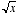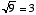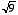index: click on a letter A B C D E F G H I J K L M N O P Q R S T U V W X Y Z A to Z index index: subject areas numbers & symbols sets, logic, proofs geometry algebra trigonometry advanced algebra & pre-calculus calculus advanced topics probability & statistics real world applications multimedia entrieswww.mathwords.com about mathwords website feedback

 Square Root A nonnegative number that must be multiplied times itself to equal a given number. The square root of x is writtenor x½. For example,since 32 = 9. Note:never refers to a negative number. Even though (–3)(–3) = 9, we do not say that –3 is a value of. Also, if x itself is negative thenis imaginary.     See also